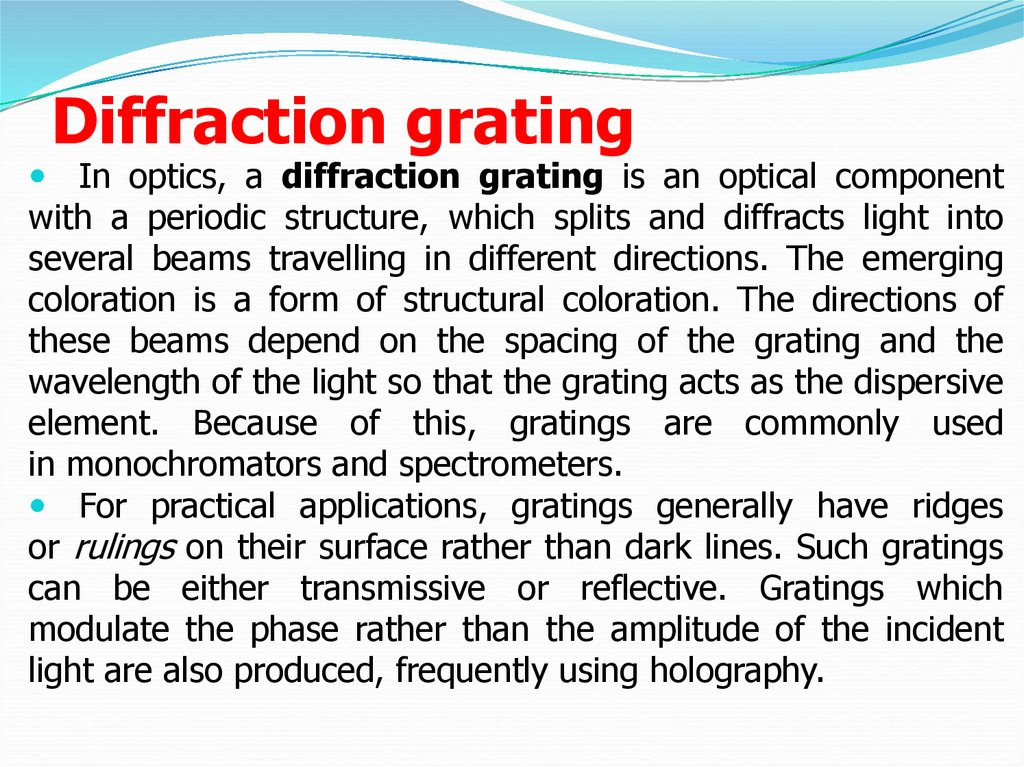# Diffraction grating

## 2. Diffraction grating

In optics, a diffraction grating is an optical component
with a periodic structure, which splits and diffracts light into
several beams travelling in different directions. The emerging
coloration is a form of structural coloration. The directions of
these beams depend on the spacing of the grating and the
wavelength of the light so that the grating acts as the dispersive
element. Because of this, gratings are commonly used
in monochromators and spectrometers.
For practical applications, gratings generally have ridges
or rulings on their surface rather than dark lines. Such gratings
can be either transmissive or reflective. Gratings which
modulate the phase rather than the amplitude of the incident
light are also produced, frequently using holography.

## 3.

The principles of diffraction gratings were discovered
by James Gregory, about a year after Newton's prism
experiments, initially with items such as bird feathers. The
first
diffraction
grating
was
around 1785 by Philadelphia inventor David Rittenhouse,
who strung hairs between two finely threaded screws. This
was similar to notable German physicist Joseph von
Fraunhofer's wire diffraction grating in 1821.

## 4.

The relationship between the grating spacing and the
angles of the incident and diffracted beams of light is
known as the grating equation.
According to the Huygens–Fresnel principle, each point
on the wavefront of a propagating wave can be considered
to act as a point source, and the wavefront at any
subsequent point can be found by adding together the
contributions from each of these individual point sources.
Gratings may be of the 'reflective' or 'transmissive'
type, analogous to a mirror or lens respectively. A grating
has a 'zero-order mode' (where m = 0), in which there is
no diffraction and a ray of light behaves according to the
laws of reflection and refraction the same as with a mirror
or lens respectively.

## 5.

An idealised grating is considered here which is made up of a set of slits of
spacing d, that must be wider than the wavelength of interest to cause diffraction.
Assuming
a
plane
wave
of
monochromatic
light
of
wavelength λ with normal incidence (perpendicular to the grating), each slit in the
grating acts as a quasi point-source from which light propagates in all directions
(although this is typically limited to a hemisphere). After light interacts with the
grating, the diffracted light is composed of the sum of interfering wave components
emanating from each slit in the grating. At any given point in space through which
diffracted light may pass, the path length to each slit in the grating will vary. Since
the path length varies, generally, so will the phases of the waves at that point from
each of the slits, and thus will add or subtract from one another to create peaks and
valleys, through the phenomenon of additive and destructive interference. When the
path difference between the light from adjacent slits is equal to half the
wavelength, λ/2, the waves will all be out of phase, and thus will cancel each other to
create points of minimum intensity. Similarly, when the path difference is λ, the
phases will add together and maxima will occur. The maxima occur at angles θm,
which satisfy the relationship d sinθm/λ = | m |, where θm is the angle between the
diffracted ray and the grating's normal vector, and d is the distance from the center
of one slit to the center of the adjacent slit, and m is an integer representing the
propagation-mode of interest.